Statement Questions - Division

Chapter 1 Class 7 Integers
Concept wise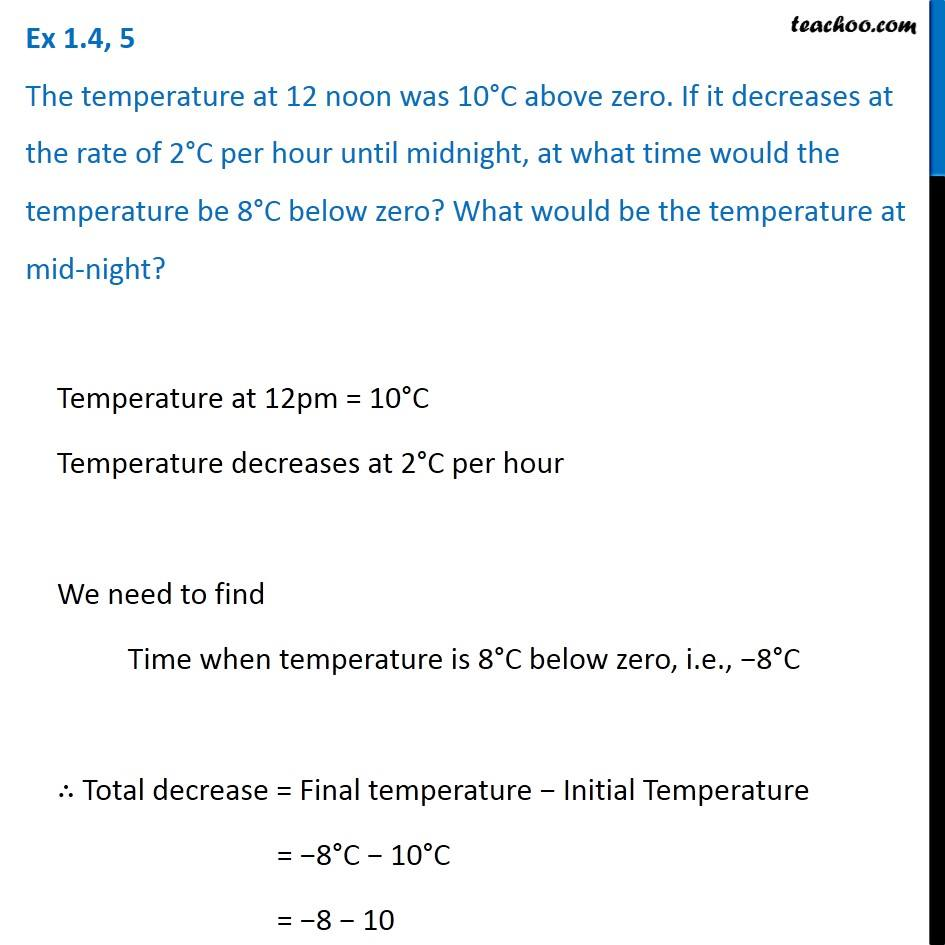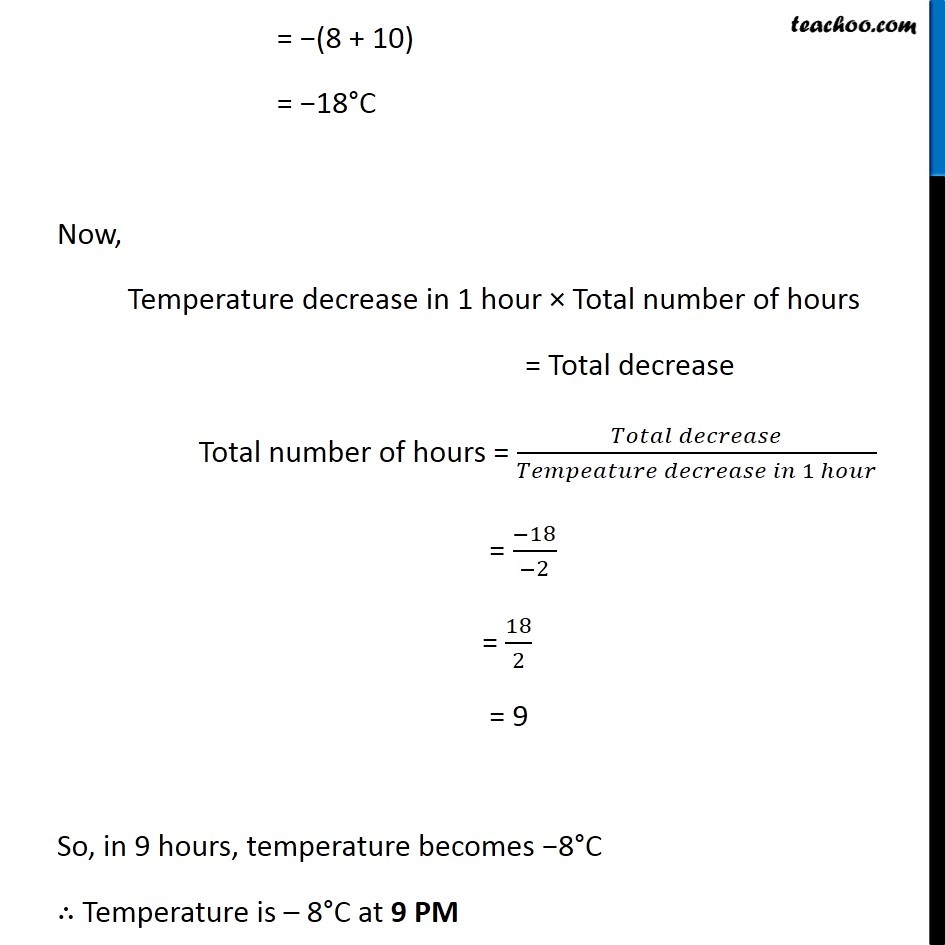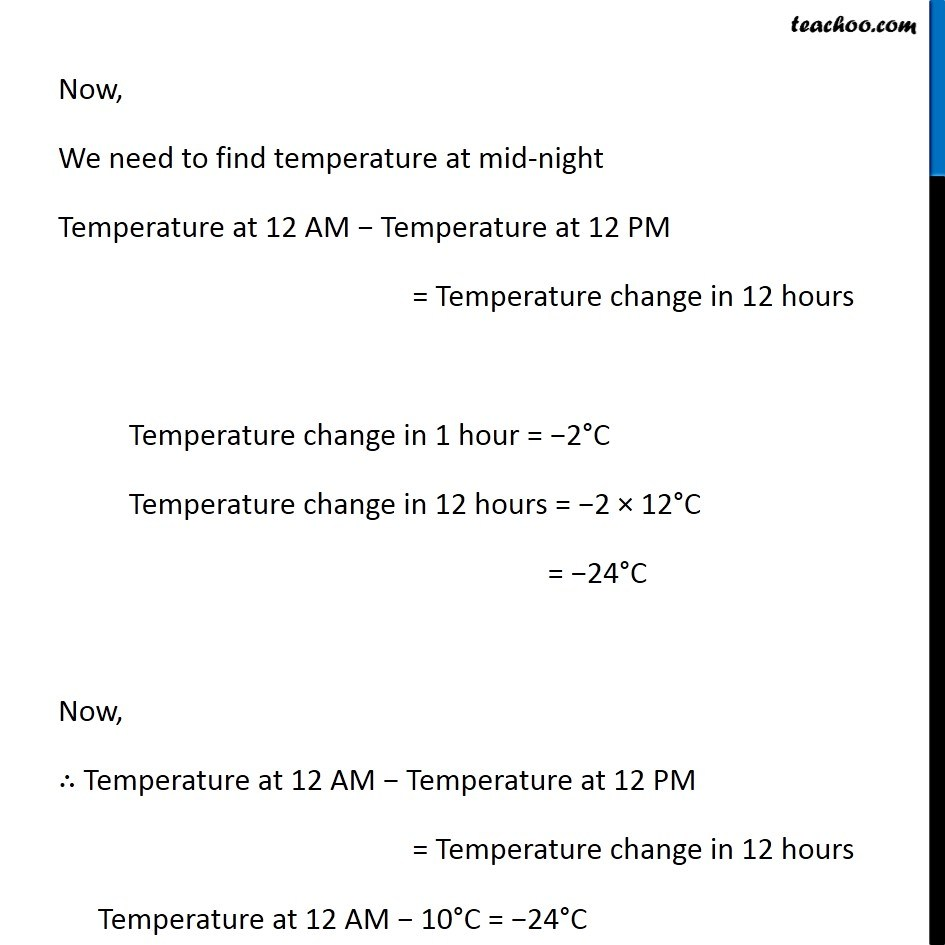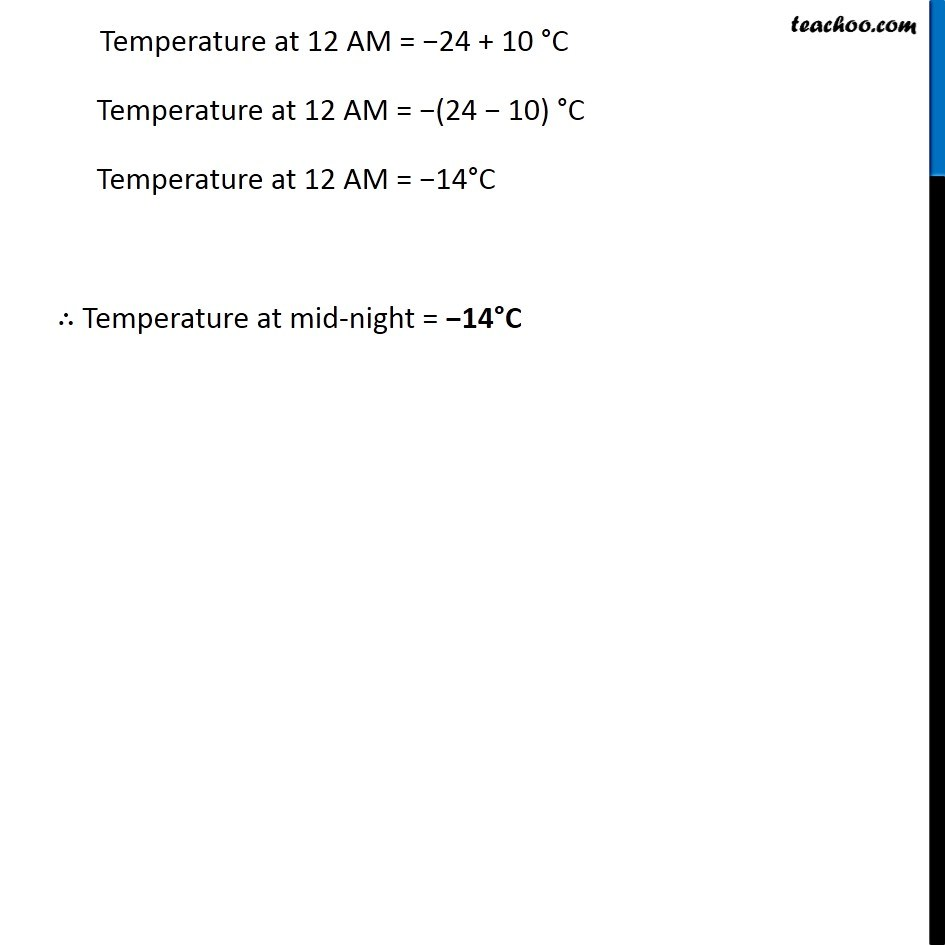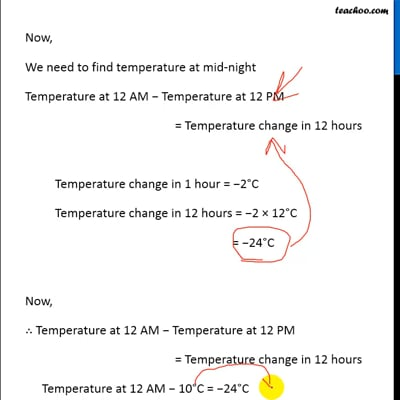This video is only available for Teachoo black users

### Transcript

Ex 1.4, 5 The temperature at 12 noon was 10°C above zero. If it decreases at the rate of 2°C per hour until midnight, at what time would the temperature be 8°C below zero? What would be the temperature at mid-night?Temperature at 12pm = 10°C Temperature decreases at 2°C per hour We need to find Time when temperature is 8°C below zero, i.e., −8°C ∴ Total decrease = Final temperature − Initial Temperature = −8°C − 10°C = −8 − 10 = −(8 + 10) = −18°C Now, Temperature decrease in 1 hour × Total number of hours = Total decrease Total number of hours = (𝑇𝑜𝑡𝑎𝑙 𝑑𝑒𝑐𝑟𝑒𝑎𝑠𝑒)/(𝑇𝑒𝑚𝑝𝑒𝑎𝑡𝑢𝑟𝑒 𝑑𝑒𝑐𝑟𝑒𝑎𝑠𝑒 𝑖𝑛 1 ℎ𝑜𝑢𝑟) = (−18)/(−2) = 18/2 = 9 So, in 9 hours, temperature becomes −8°C ∴ Temperature is – 8°C at 9 PM Now, We need to find temperature at mid-night Temperature at 12 AM − Temperature at 12 PM = Temperature change in 12 hours Temperature change in 1 hour = −2°C Temperature change in 12 hours = −2 × 12°C = −24°C Now, ∴ Temperature at 12 AM − Temperature at 12 PM = Temperature change in 12 hours Temperature at 12 AM − 10°C = −24°C Temperature at 12 AM = −24 + 10 °C Temperature at 12 AM = −(24 − 10) °C Temperature at 12 AM = −14°C ∴ Temperature at mid-night = −14°C